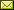## Quantum Set Theory Extending the Standard Probabilistic Interpretation of Quantum Theory (Extended Abstract)

 Masanao Ozawa (Nagoya University)

 The notion of equality between two observables will play many important roles in foundations of quantum theory. However, the standard probabilistic interpretation based on the conventional Born formula does not give the probability of equality relation for a pair of arbitrary observables, since the Born formula gives the probability distribution only for a commuting family of observables. In this paper, quantum set theory developed by Takeuti and the present author is used to systematically extend the probabilistic interpretation of quantum theory to define the probability of equality relation for a pair of arbitrary observables. Applications of this new interpretation to measurement theory are discussed briefly.

Invited Presentation in Bob Coecke, Ichiro Hasuo and Prakash Panangaden: Proceedings of the 11th workshop on Quantum Physics and Logic (QPL 2014), Kyoto, Japan, 4-6th June 2014, Electronic Proceedings in Theoretical Computer Science 172, pp. 15–26.
Published: 28th December 2014.

 ArXived at: http://dx.doi.org/10.4204/EPTCS.172.2 bibtex PDF
References in reconstructed bibtex, XML and HTML format (approximated).
 Comments and questions to:eptcs@eptcs.org For website issues:webmaster@eptcs.org